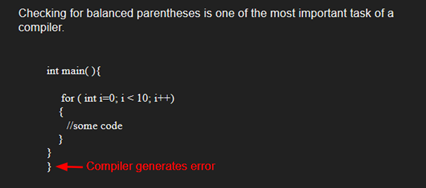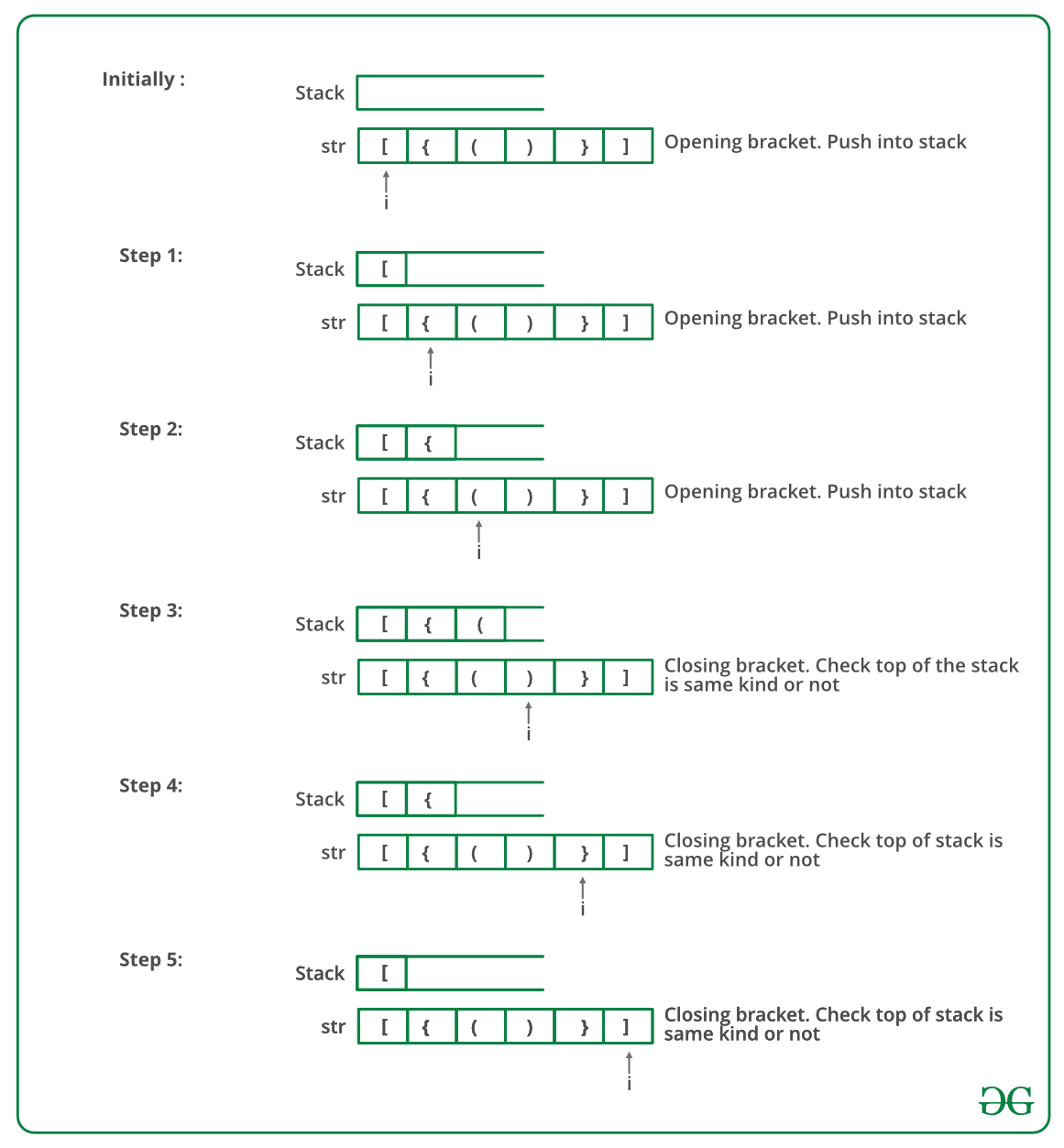# C Program To Check For Balanced Brackets In An Expression (Well-Formedness) Using Stack

• Last Updated : 14 Dec, 2021

Given an expression string exp, write a program to examine whether the pairs and the orders of “{“, “}”, “(“, “)”, “[“, “]” are correct in exp.

Example

Input: exp = “[()]{}{[()()]()}”
Output: Balanced

Input: exp = “[(])”
Output: Not BalancedAlgorithm:

• Declare a character stack S.
• Now traverse the expression string exp.
1. If the current character is a starting bracket (‘(‘ or ‘{‘ or ‘[‘) then push it to stack.
2. If the current character is a closing bracket (‘)’ or ‘}’ or ‘]’) then pop from stack and if the popped character is the matching starting bracket then fine else brackets are not balanced.
• After complete traversal, if there is some starting bracket left in stack then “not balanced”

Below image is a dry run of the above approach:Below is the implementation of the above approach:

## C

 `#include ``#include ``#define bool int`` ` `// structure of a stack node``struct` `sNode {``    ``char` `data;``    ``struct` `sNode* next;``};`` ` `// Function to push an item to stack``void` `push(``struct` `sNode** top_ref, ``int` `new_data);`` ` `// Function to pop an item from stack``int` `pop(``struct` `sNode** top_ref);`` ` `// Returns 1 if character1 and character2 are matching left``// and right Brackets``bool` `isMatchingPair(``char` `character1, ``char` `character2)``{``    ``if` `(character1 == ``'('` `&& character2 == ``')'``)``        ``return` `1;``    ``else` `if` `(character1 == ``'{'` `&& character2 == ``'}'``)``        ``return` `1;``    ``else` `if` `(character1 == ``'['` `&& character2 == ``']'``)``        ``return` `1;``    ``else``        ``return` `0;``}`` ` `// Return 1 if expression has balanced Brackets``bool` `areBracketsBalanced(``char` `exp``[])``{``    ``int` `i = 0;`` ` `    ``// Declare an empty character stack``    ``struct` `sNode* stack = NULL;`` ` `    ``// Traverse the given expression to check matching``    ``// brackets``    ``while` `(``exp``[i]) ``    ``{``        ``// If the exp[i] is a starting bracket then push``        ``// it``        ``if` `(``exp``[i] == ``'{'` `|| ``exp``[i] == ``'('` `|| ``exp``[i] == ``'['``)``            ``push(&stack, ``exp``[i]);`` ` `        ``// If exp[i] is an ending bracket then pop from``        ``// stack and check if the popped bracket is a``        ``// matching pair*/``        ``if` `(``exp``[i] == ``'}'` `|| ``exp``[i] == ``')'``            ``|| ``exp``[i] == ``']'``) {`` ` `            ``// If we see an ending bracket without a pair``            ``// then return false``            ``if` `(stack == NULL)``                ``return` `0;`` ` `            ``// Pop the top element from stack, if it is not``            ``// a pair bracket of character then there is a``            ``// mismatch.``            ``// his happens for expressions like {(})``            ``else` `if` `(!isMatchingPair(pop(&stack), ``exp``[i]))``                ``return` `0;``        ``}``        ``i++;``    ``}`` ` `    ``// If there is something left in expression then there``    ``// is a starting bracket without a closing``    ``// bracket``    ``if` `(stack == NULL)``        ``return` `1; ``// balanced``    ``else``        ``return` `0; ``// not balanced``}`` ` `// Driver code``int` `main()``{``    ``char` `exp`` = ``"{()}[]"``;`` ` `    ``// Function call``    ``if` `(areBracketsBalanced(``exp``))``        ``printf``("Balanced ``");``    ``else``        ``printf``("Not Balanced ``");``    ``return` `0;``}`` ` `// Function to push an item to stack``void` `push(``struct` `sNode** top_ref, ``int` `new_data)``{``    ``// allocate node``    ``struct` `sNode* new_node``        ``= (``struct` `sNode*)``malloc``(``sizeof``(``struct` `sNode));`` ` `    ``if` `(new_node == NULL) {``        ``printf``(``"Stack overflow n"``);``        ``getchar``();``        ``exit``(0);``    ``}`` ` `    ``// put in the data``    ``new_node->data = new_data;`` ` `    ``// link the old list off the new node``    ``new_node->next = (*top_ref);`` ` `    ``// move the head to point to the new node``    ``(*top_ref) = new_node;``}`` ` `// Function to pop an item from stack``int` `pop(``struct` `sNode** top_ref)``{``    ``char` `res;``    ``struct` `sNode* top;`` ` `    ``// If stack is empty then error``    ``if` `(*top_ref == NULL) {``        ``printf``(``"Stack overflow n"``);``        ``getchar``();``        ``exit``(0);``    ``}``    ``else` `{``        ``top = *top_ref;``        ``res = top->data;``        ``*top_ref = top->next;``        ``free``(top);``        ``return` `res;``    ``}``}`
Output
`Balanced`

Time Complexity: O(n)
Auxiliary Space: O(n) for stack.

Please refer complete article on Check for Balanced Brackets in an expression (well-formedness) using Stack for more details!

My Personal Notes arrow_drop_up